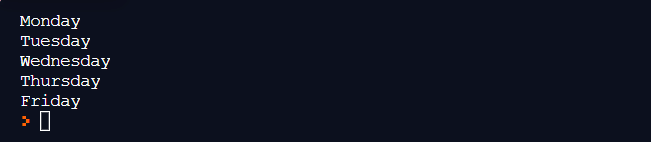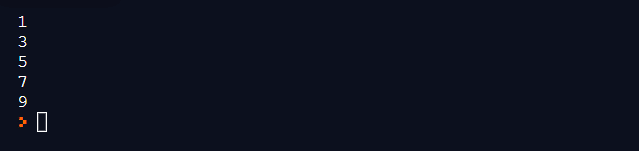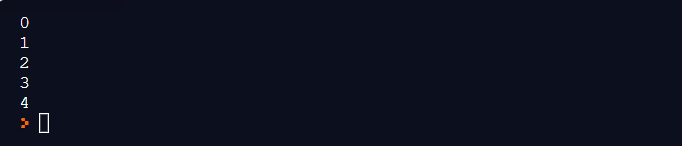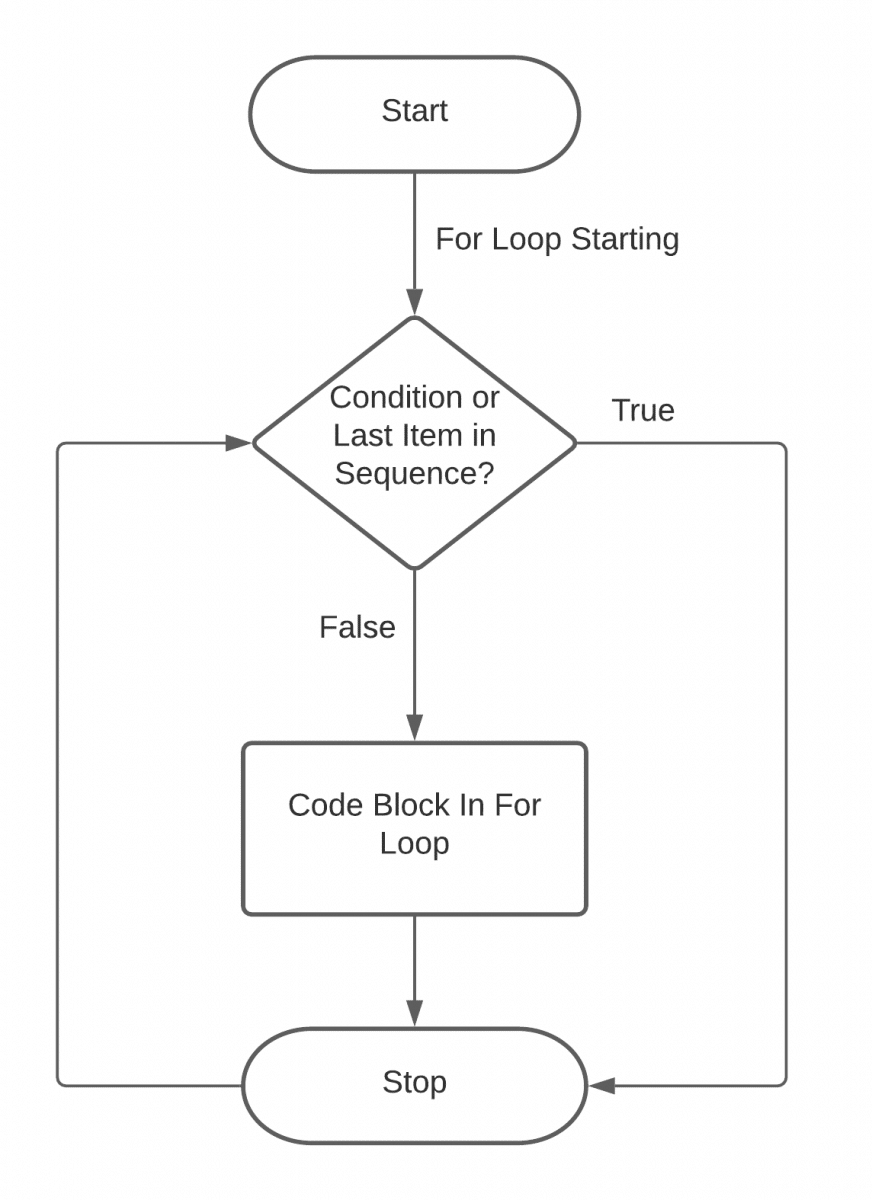The concept of "loops" in programming reminds me of my third-grade teacher teaching me about multiplication. She stated the importance of multiplication to avoid repetitive additions, followed by an example of 2+2+2 = 2*3. While everything else might have faded away, "avoiding repetitive tasks" stayed with me and helped in computer science a lot. Python "for" loops is a no different phenomenon. Python "for" loops is a way to avoid repetitive tasks described inside the loop which we will see later in this chapter. In this post, I will try to put down a solid foundation of loops in Python as they are used very exhaustively in programming. On the same lines, let me mention the main highlights of this post:

• What is Python "for" Loop?
• How to implement "for" loops in Python?
• Implementing "for" loop in Python.
• Implementing "for" Loops using "range" function.
• Flowchart for Python "for" loop.
• Nested "for" loops in Python.

## What is Python "for" loop?

The "for" loop in Python is a way to run a repetitive block of code traversing over a sequence of any kind. The sequence can be a list, a python dictionary, a string, a range of numbers, etc. If you are pondering over "when should I use "for" loops in Python" now or during your PCEP exam, think the before-said statement as your answer. When you are thinking about iterations and running a block of code repeatedly, a loop is your way to go!

The "for" loop will run until all the items in the sequence (or any other iterable object) are not traversed completely. Although there is an exception of the "break" statement which can terminate the loop mid-way. But naturally, it will traverse the sequence completely.

The structure of the python "for" loop is very simple:

``````for var in sequence:

//
``````

The "var" in the structure is the variable name that will take the current item's value from the sequence. The "sequence" is the variable denoting the sequence name. Don't worry if things are not clear now, they will be when we implement the "for" loop in Python practically.

It is important to note that Python "for" loop is a bit different than the "for" loop of other programming languages. In other programming languages, a "for" loop initiates a variable with a value (although this is optional but standard ), performs condition checks, and then performs a specified operation on every iteration of the loop. The operation is more commonly used as "var++" denoting increment in var value by 1.

In Python though, the "for" loop works more like an iterator that iterates over a given sequence or iterable object and the variable taking value from that sequence. In the next section, we shall implement the "for" loop which will help clear out the picture.

## How to implement "for" loops in Python?

To implement the "for" loop in Python, we will pick up the same structure and use that as our skeleton in the program. In this section we will go through two examples: one by implementing the "for" loop using a list as a sequence and implementing the "for" loop in range.

### Implementing "for" loop in Python

Before writing our loop code, we need to create a sequence. Let's use a list which contains the name of the days in the week. The initialization of the list is as follows:

``````days = ["Monday", "Tuesday", "Wednesday", "Thursday", "Friday"]
``````

Note: The above code uses "Lists in Python" which is covered in the later chapters.

Now that we have our sequence ready, we can iterate over it. There is no prior declaration required for the variable we use inside the "for" loop. The following python "for" loop program iterates over the list and prints the list elements as output:

``````days = ["Monday", "Tuesday", "Wednesday", "Thursday", "Friday"]
for day in days:
print (day)
``````

In the above program, the variable "day" will take values from "days" one by one until the whole list is not traversed. Like other programming languages, there is no need to subscript the variable as days[day]. Python takes care of that itself.

Execute the above code to produce the following output:I hope it was easy enough! We will take one more example, a little bit different, to understand the basics of "for" loop more deeply.

### Implementing "for" loops using "range" function

In this example, we will iterate over a series of numbers using the "range" method. The "range" method defines a range of numbers in a continuous or non-continuous fashion. The "range" method in Python can also work backward and its working closely resembles the "for" loop of other languages. Since the "range" method works as an iterator over numbers, it can accept only integers as a parameter.

The syntax of python "for" loop with range method is as follows:

``````for var in range(min, max, step):
//code
``````

The loop above will run from the min value to the max - 1 value with an increment of step to the variable "var". So the following would be the iteration:

``````iteration 1: var = min

iteration 2: var = min + 1 (since step = 1)

iteration 3: var = min + 2

....

iteration max - 1: var = min + max - 1
``````

The following example will print the numbers from 1 to 10 with an increment of 2.

``````for i in range(1, 10, 2):
print (i)
``````

The above code will produce the following output:The following points will come in handy while working with the range method:

• A negative step means a decrement in range. Therefore, step as -2 will decrement the value by 2.
• If the minimum value is 0, you can omit the min value part and write the maximum value directly as the default min value is 1.
• If the step is 1, there is no need to write the step value as the default step value is 1.

#### Python "range" function with a single parameter

In the wake of the above-said points in the bullets, a small example will help you understand how does python "for" loop works with the "range" function with a single parameter.

The following code uses a single parameter in the "range" function:

``````for i in range(5):
print(i)
``````

With a single value, the range function takes it as a "max" value considering 0 as the default minimum and 1 as the default step value. The "for" loop would execute as follows:The output is in accordance with our assumptions and considers 0 as the minimum value with 1 as the default step.

#### Python "range" function with two parameters

Similar to the above section, the python "for" loop will run with the default step as 1 when we have given only two parameters. They will be considered as a minimum value and maximum value respectively.

The following for loop in Python uses a range function with two parameters:

``````for i in range(5,10):
print(i)
``````

The output of the above code is as follows:You must have observed how python skipped the "max" value inside the range function. Therefore, remember that python "for" loop with the range function executes from min to max - 1 value.

### Flowchart for Python "for" loop

The "for" loop in Python can also be visualized with a flowchart which creates a better picture of the overall flow. The following image describes the flowchart for the same.I hope it comes in handy while practicing for the "for" loop in Python. As a next step, we will try to run one "for" loop inside another. This is also termed "nested looping".

### Nested "For" Loops in Python

The word "nested" denotes one thing inside another. Similarly, nested loops mean when we code a loop inside another loop in any programming language. They are a simple concept where the inside loop can be considered the code block of the outside loop as follows:

``````for i in range(1,10):
for j in range(1, 10):
//code
``````

Can you determine the output after implementing the above code?

In the above code, the outside "for" loop runs from 1 to 9 while the inside loop will run 9 times for each iteration of the outer loop.  So, for example:

Iteration 1:

``````i = 1, j = 1

i = 1, j = 2

i = 1, j = 3

...

i = 9, j = 9

``````

Edit the above-given code as follows:

``````for i in range(1,10):
for j in range(1, 10):
print("i = " + str(i) + " j = " + str(j))

``````

Run the above code to see the following output:Note: The above screenshot is the partial output as the printed list is very long.

Also, the nested loops do not necessarily be two loops. You can implement any number of nested loops one inside another but as a programmer, I must suggest that nested loops should be the last option in programming as they increase the time complexity of the program by the power.

Alright, this was all about the "for" loop in Python. In some programming languages, you might find another sister loop of the "for" loop known as "for each" loop. In Python, we do not have the for-each loop but all its traversal functionalities are covered through the "for" loop only. Therefore, it is easier to loop things in Python.

Another important loop in Python from programming as well as PCEP point of view is the "while" loop. In the next section, we shall go through the "while" loop and see how it differs from the "for" loop in Python.

## Key Takeaways

• Loops in Python help avoid repeatable tasks confining them to a few lines of code.
• The "for loop" in Python is one of the loops built in the language.
• The "for" loops in Python are very versatile and can iterate over a sequence, list, dictionary, or any iterable object.
• A "for" loop inside another "for" loop is called a nested "for" loop.ReviewersLakshay Sharma